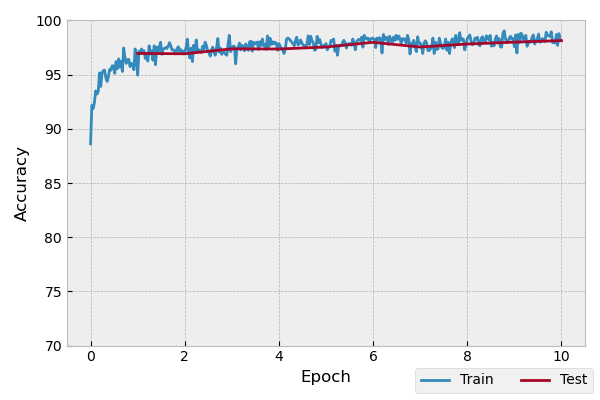# Model-Agnostic Meta-Learning

Meta-reinforcement learning has achieved significant successes in various applications. Model-Agnostic Meta-Learning (MAML) [FAL17] is the pioneer one. In this tutorial, we will show how to train MAML on few-shot Omniglot classification with TorchOpt step by step. The full script is at examples/few-shot/maml_omniglot.py.

Contrary to existing differentiable optimizer libraries such as higher, which follows the PyTorch designing which leads to inflexible API, TorchOpt provides an easy way of construction through the code-level.

## Overview

There are six steps to finish MAML training pipeline:

2. Build the Network: build the neural network architecture of model;

3. Train: meta-train;

4. Test: meta-test;

5. Plot: plot the results;

6. Pipeline: combine step 3-5 together;

In the following sections, we will set up Load Dataset, build the neural network, train-test, and plot to successfully run the MAML training and evaluation pipeline. Here is the overall procedure:

In your Python code, simply import torch and load the dataset, the full script is at examples/few-shot/support/omniglot_loaders.py:

from .support.omniglot_loaders import OmniglotNShot
import torch

device = torch.device('cuda:0')
db = OmniglotNShot(
'/tmp/omniglot-data',
n_way=args.n_way,
k_shot=args.k_spt,
k_query=args.k_qry,
imgsz=28,
rng=rng,
device=device,
)


The goal is to train a model for few-shot Omniglot classification.

## Build the Network

TorchOpt supports any user-defined PyTorch networks. Here is an example:

import torch, numpy as np
from torch import nn
import torch.optim as optim

net = nn.Sequential(
nn.Conv2d(1, 64, 3),
nn.BatchNorm2d(64, momentum=1.0, affine=True),
nn.ReLU(inplace=False),
nn.MaxPool2d(2, 2),
nn.Conv2d(64, 64, 3),
nn.BatchNorm2d(64, momentum=1.0, affine=True),
nn.ReLU(inplace=False),
nn.MaxPool2d(2, 2),
nn.Conv2d(64, 64, 3),
nn.BatchNorm2d(64, momentum=1.0, affine=True),
nn.ReLU(inplace=False),
nn.MaxPool2d(2, 2),
nn.Flatten(),
nn.Linear(64, args.n_way),
).to(device)

# We will use Adam to (meta-)optimize the initial parameters


## Train

Define the train function:

def train(db, net, meta_opt, epoch, log):
net.train()
n_train_iter = db.x_train.shape // db.batchsz
inner_opt = torchopt.MetaSGD(net, lr=1e-1)

for batch_idx in range(n_train_iter):
start_time = time.time()
# Sample a batch of support and query images and labels.
x_spt, y_spt, x_qry, y_qry = db.next()

# TODO: Maybe pull this out into a separate module so it
# doesn't have to be duplicated between train and test?

# Initialize the inner optimizer to adapt the parameters to
# the support set.
n_inner_iter = 5

qry_losses = []
qry_accs = []

net_state_dict = torchopt.extract_state_dict(net)
optim_state_dict = torchopt.extract_state_dict(inner_opt)
# Optimize the likelihood of the support set by taking
# gradient steps w.r.t. the model's parameters.
for _ in range(n_inner_iter):
spt_logits = net(x_spt[i])
spt_loss = F.cross_entropy(spt_logits, y_spt[i])
inner_opt.step(spt_loss)

# The final set of adapted parameters will induce some
# final loss and accuracy on the query dataset.
# These will be used to update the model's meta-parameters.
qry_logits = net(x_qry[i])
qry_loss = F.cross_entropy(qry_logits, y_qry[i])
qry_acc = (qry_logits.argmax(dim=1) == y_qry[i]).float().mean()
qry_losses.append(qry_loss)
qry_accs.append(qry_acc.item())

torchopt.recover_state_dict(net, net_state_dict)
torchopt.recover_state_dict(inner_opt, optim_state_dict)

qry_losses = torch.mean(torch.stack(qry_losses))
qry_losses.backward()
meta_opt.step()
qry_losses = qry_losses.item()
qry_accs = 100.0 * np.mean(qry_accs)
i = epoch + float(batch_idx) / n_train_iter
iter_time = time.time() - start_time

print(
f'[Epoch {i:.2f}] Train Loss: {qry_losses:.2f} | Acc: {qry_accs:.2f} | Time: {iter_time:.2f}'
)
log.append(
{
'epoch': i,
'loss': qry_losses,
'acc': qry_accs,
'mode': 'train',
'time': time.time(),
}
)


## Test

Define the test function:

def test(db, net, epoch, log):
# Crucially in our testing procedure here, we do *not* fine-tune
# the model during testing for simplicity.
# Most research papers using MAML for this task do an extra
# stage of fine-tuning here that should be added if you are
# adapting this code for research.
net.train()
n_test_iter = db.x_test.shape // db.batchsz
inner_opt = torchopt.MetaSGD(net, lr=1e-1)

qry_losses = []
qry_accs = []

for batch_idx in range(n_test_iter):
x_spt, y_spt, x_qry, y_qry = db.next('test')

# TODO: Maybe pull this out into a separate module so it
# doesn't have to be duplicated between train and test?
n_inner_iter = 5

net_state_dict = torchopt.extract_state_dict(net)
optim_state_dict = torchopt.extract_state_dict(inner_opt)
# Optimize the likelihood of the support set by taking
# gradient steps w.r.t. the model's parameters.
for _ in range(n_inner_iter):
spt_logits = net(x_spt[i])
spt_loss = F.cross_entropy(spt_logits, y_spt[i])
inner_opt.step(spt_loss)

# The query loss and acc induced by these parameters.
qry_logits = net(x_qry[i]).detach()
qry_loss = F.cross_entropy(qry_logits, y_qry[i])
qry_acc = (qry_logits.argmax(dim=1) == y_qry[i]).float().mean()
qry_losses.append(qry_loss.item())
qry_accs.append(qry_acc.item())

torchopt.recover_state_dict(net, net_state_dict)
torchopt.recover_state_dict(inner_opt, optim_state_dict)

qry_losses = np.mean(qry_losses)
qry_accs = 100.0 * np.mean(qry_accs)

print(f'[Epoch {epoch+1:.2f}] Test Loss: {qry_losses:.2f} | Acc: {qry_accs:.2f}')
log.append(
{
'epoch': epoch + 1,
'loss': qry_losses,
'acc': qry_accs,
'mode': 'test',
'time': time.time(),
}
)


## Plot

TorchOpt supports any user-defined PyTorch networks and optimizers. Yet, of course, the inputs and outputs must comply with TorchOpt’s API. Here is an example:

def plot(log):
# Generally you should pull your plotting code out of your training
# script but we are doing it here for brevity.
df = pd.DataFrame(log)

fig, ax = plt.subplots(figsize=(6, 4))
train_df = df[df['mode'] == 'train']
test_df = df[df['mode'] == 'test']
ax.plot(train_df['epoch'], train_df['acc'], label='Train')
ax.plot(test_df['epoch'], test_df['acc'], label='Test')
ax.set_xlabel('Epoch')
ax.set_ylabel('Accuracy')
ax.set_ylim(70, 100)
fig.legend(ncol=2, loc='lower right')
fig.tight_layout()
fname = 'maml-accs.png'
print(f'--- Plotting accuracy to {fname}')
fig.savefig(fname)
plt.close(fig)


## Pipeline

We can now combine all the components together, and plot the results.

log = []
for epoch in range(10):
train(db, net, meta_opt, epoch, log)
test(db, net, epoch, log)
plot(log)References

FAL17

Chelsea Finn, Pieter Abbeel, and Sergey Levine. Model-agnostic meta-learning for fast adaptation of deep networks. In Doina Precup and Yee Whye Teh, editors, Proceedings of the 34th International Conference on Machine Learning, ICML 2017, Sydney, NSW, Australia, 6-11 August 2017, volume 70 of Proceedings of Machine Learning Research, 1126–1135. PMLR, 2017. URL: http://proceedings.mlr.press/v70/finn17a.html.